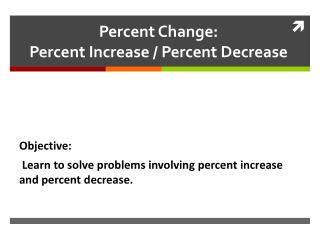DownloadDownload PresentationPercent Change: Percent Increase / Percent Decrease

# Percent Change: Percent Increase / Percent Decrease

Télécharger la présentation## Percent Change: Percent Increase / Percent Decrease

- - - - - - - - - - - - - - - - - - - - - - - - - - - E N D - - - - - - - - - - - - - - - - - - - - - - - - - - -
##### Presentation Transcript

1. Percent Change: Percent Increase / Percent Decrease Objective: Learn to solve problems involving percent increase and percent decrease.

2. Gaby went to Kalas and wanted to buy a really cool red dress. The dress was on sale 25% off. If the original price of the dress was \$165 dollars, how much will the price be reduced? • When a price or number changes, you can use a formula to describe the increase or decrease.

3. The Formula is… • Percent change = amount of change 100 original amount So you have to substitute the values…. 25 = X 100 \$165 100 X = 4125 100 100 X = 41.25

4. Example 2: • Find the percent increase or decrease, if 15 is increased to 20. Percent change = amount of change 100 original amount P = 5 100 15 15 P = 500 15 15 P = 33.3%

5. Example 3: • Find the amount of increase or decrease if 75 is increased by 40%. Percent change = amount of change 100 original amount 40 = X 100 75 100 X = 3000 X = 30

6. Your Example: Find the percent of increase or decrease if 96 is decreased to 72. • Percent change = amount of change 100 original amount

7. Homework: Find each percent increase or decrease. Find the amount of increase or decrease. 58 is increased by 72%. 28 is increased by 150%. 506 is decreased by 57%. 4 is increased by 12%. 48 is increased by 35%. • 125 is decreased to 2. • 360 is increased to 361. • 84 is decreased to 28. • 615 is decreased to 450. • 460 is increased to 500.